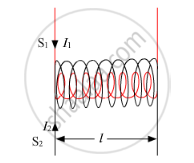# Derive an Expression for the Mutual Inductance of Two Long Co-axial Solenoids of Same Length Wound One Over the Other - Physics

Derive an expression for the mutual inductance of two long co-axial solenoids of same length wound one over the other,

Obtain the expression for the the mutual inductance of two long co-axial solenoids S1 and S2 wound one over the other, each of length L and radii r1 and r2 and n1 and n2 number of turns per unit length, when a current I is set up in the outer solenoid S2.

#### Solution

Consider two long solenoids S1 and S2 of same length (l ) such that solenoid S2 surrounds solenoid S1 completely.Let:

n1 = Number of turns per unit length of S1

n2 = Number of turns per unit length of S2

I1 = Current passed through solenoid S1

Φ21 = Flux linked with S2 due to current flowing through S1

Φ21I1

Φ21 = M21I1

Where

M21 = Coefficient of mutual induction of the two solenoids

When current is passed through solenoid S1, an emf is induced in solenoid S2.

Magnetic field produced inside solenoid S1 on passing current through it is given by

B1 = μ0n1I1

Magnetic flux linked with each turn of solenoid S2 will be equal to B1 times the area of cross-section of solenoid S1

Magnetic flux linked with each turn of the solenoid S2 = B1A

Therefore, total magnetic flux linked with the solenoid S2 is given by

Φ21 = B1A × n2l = μ0n1I1 × A× n2l

Φ21 = μ0n1n2AI1

∴ M21 = μ0n1n2Al

Similarly, the mutual inductance between the two solenoids, when current is passed through solenoid S2 and induced emf is produced in solenoid S1, is given by

M12 = μ0n1n2Al

∴ M12 = M21 = M (say)

Hence, coefficient of mutual induction between the two long solenoids is given by

M=μ0n1n2Al

Concept: Solenoid and the Toroid - the Solenoid
Is there an error in this question or solution?
2014-2015 (March) Delhi Set 1

Share